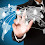# PHP Login Register Script by using AngularJS

In this tutorial, We will learn How to make PHP Login Register System by using AngularJS. Here we make simple login and register page by using AngularJS. We all know AngularJS is a javascript framework and this library has been written in pure javascript and it is developed and manage by Google. So, in Web development using of AngularJS has been increase. So, many web developers has been use AngularJS for their web development as front-end. For this here we have make this post in which we have describe how to make AngularJS Login Register page by using PHP.

Login and Register is a entry point of any website. So, if we want to access any web system feature then we want to make first registration and after registration we can login into system. So, Login and Registration is a required functionality of any web application. For this here we have make discuss how to create login and registration page in angularjs with PHP and Mysql. This tutorial will useful to AngularJS beginner tutorial becuase here we have discuss PHP Login Register Script by using AngularJS from scratch.

### index.php

``````
<?php

//index.php

session_start();

?>
<!DOCTYPE html>
<html>
<title>AngularJS Register Login Script using PHP Mysql</title>
<style>
.form_style
{
width: 600px;
margin: 0 auto;
}
</style>
<body>
<br />
<h3 align="center">AngularJS Register Login Script using PHP Mysql</h3>
<br />

<?php
if(!isset(\$_SESSION["name"]))
{
?>
<a href="#" class="close" ng-click="closeMsg()" aria-label="close">&times;</a>
</div>

</div>
<div class="panel-body">
<div class="form-group">
<input type="text" name="email" ng-model="loginData.email" class="form-control" />
</div>
<div class="form-group">
</div>
<div class="form-group" align="center">
<br />
</div>
</form>
</div>
</div>

<div class="panel panel-default" ng-show="register_form">
<h3 class="panel-title">Register</h3>
</div>
<div class="panel-body">
<form method="post" ng-submit="submitRegister()">
<div class="form-group">
<input type="text" name="name" ng-model="registerData.name" class="form-control" />
</div>
<div class="form-group">
<input type="text" name="email" ng-model="registerData.email" class="form-control" />
</div>
<div class="form-group">
</div>
<div class="form-group" align="center">
<input type="submit" name="register" class="btn btn-primary" value="Register" />
<br />
</div>
</form>
</div>
</div>
<?php
}
else
{
?>
<div class="panel panel-default">
<h3 class="panel-title">Welcome to system</h3>
</div>
<div class="panel-body">
<h1>Welcome - <?php echo \$_SESSION["name"];?></h1>
<a href="logout.php">Logout</a>
</div>
</div>
<?php
}
?>

</div>
</body>
</html>

<script>
\$scope.closeMsg = function(){
};

\$scope.showRegister = function(){
\$scope.register_form = true;
};

\$scope.register_form = false;
};

\$scope.submitRegister = function(){
\$http({
method:"POST",
url:"register.php",
data:\$scope.registerData
}).success(function(data){
if(data.error != '')
{
}
else
{
\$scope.registerData = {};
}
});
};

\$http({
method:"POST",
}).success(function(data){
if(data.error != '')
{
}
else
{
}
});
};

});
</script>
```
```

### database_connection.php

``````
<?php

//database_connection.php

\$connect = new PDO("mysql:host=localhost;dbname=testing", "root", "");

?>
```
```

### register.php

``````
<?php

//register.php

include('database_connection.php');

\$form_data = json_decode(file_get_contents("php://input"));

\$message = '';
\$validation_error = '';

if(empty(\$form_data->name))
{
\$error[] = 'Name is Required';
}
else
{
\$data[':name'] = \$form_data->name;
}

if(empty(\$form_data->email))
{
\$error[] = 'Email is Required';
}
else
{
if(!filter_var(\$form_data->email, FILTER_VALIDATE_EMAIL))
{
\$error[] = 'Invalid Email Format';
}
else
{
\$data[':email'] = \$form_data->email;
}
}

{
}
else
{
}

if(empty(\$error))
{
\$query = "
";
\$statement = \$connect->prepare(\$query);
if(\$statement->execute(\$data))
{
\$message = 'Registration Completed';
}
}
else
{
\$validation_error = implode(", ", \$error);
}

\$output = array(
'error'  => \$validation_error,
'message' => \$message
);

echo json_encode(\$output);

?>
```
```

``````
<?php

include('database_connection.php');

session_start();

\$form_data = json_decode(file_get_contents("php://input"));

\$validation_error = '';

if(empty(\$form_data->email))
{
\$error[] = 'Email is Required';
}
else
{
if(!filter_var(\$form_data->email, FILTER_VALIDATE_EMAIL))
{
\$error[] = 'Invalid Email Format';
}
else
{
\$data[':email'] = \$form_data->email;
}
}

{
}

if(empty(\$error))
{
\$query = "
SELECT * FROM register
WHERE email = :email
";
\$statement = \$connect->prepare(\$query);
if(\$statement->execute(\$data))
{
\$result = \$statement->fetchAll();
if(\$statement->rowCount() > 0)
{
foreach(\$result as \$row)
{
{
\$_SESSION["name"] = \$row["name"];
}
else
{
}
}
}
else
{
\$validation_error = 'Wrong Email';
}
}
}
else
{
\$validation_error = implode(", ", \$error);
}

\$output = array(
'error' => \$validation_error
);

echo json_encode(\$output);

?>
```
```

### logout.php

``````
<?php

//logout.php

session_start();

session_destroy();

?>
```
```

### Database

``````
--
-- Database: `testing`
--

-- --------------------------------------------------------

--
-- Table structure for table `register`
--

CREATE TABLE `register` (
`id` int(11) NOT NULL,
`name` varchar(250) NOT NULL,
`email` varchar(250) NOT NULL,
) ENGINE=InnoDB DEFAULT CHARSET=latin1;

--
-- Indexes for dumped tables
--

--
-- Indexes for table `register`
--
ALTER TABLE `register`

--
-- AUTO_INCREMENT for dumped tables
--

--
-- AUTO_INCREMENT for table `register`
--
ALTER TABLE `register`
MODIFY `id` int(11) NOT NULL AUTO_INCREMENT;
COMMIT;
```
```

1.2.it is showing wrong password only

3.solid ... its working fine thank you for your script and demo

4.5.6.Excellent PHP example with Angular, Thank you

7.Excellent PHP example with Angular, Thank you

8.es muy claro el ejemplo gracias

9.how to connect this one with my cpanel

10.angular tutorial with php to simulate a supermarket box?

11.It allways shows me the error:
name is Required, Email is Required, Password is Required

1.did u got the solution for this

12.This is a really good script. However, is there a way i can check if a username or email already exists?
Thanks

13.14.15.